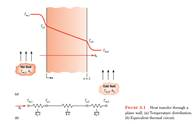Create an Account

Home / Questions / The one dimensional plane wall of Figure 31 is of thickness L 75 mm and thermal conductivity

The one dimensional plane wall of Figure 31 is of thickness L 75 mm and thermal conductivity

The one-dimensional plane wall of Figure 3.1 is of thickness L = 75 mm and thermal conductivity k = 5 W/m∙ K. The fluid temperatures are T,1 = 200°C and T∞,2 = 100°C, respectively. Using the minimum and maximum typical values of the convection heat transfer coefficients listed in Table 1.1, determine the minimum and maximum steady-state heat fluxes through the wall for (i) free convection in gases, (ii) free convection in liquids, (iii) forced convection in gases, (iv) forced convection in liquids, and (v) convection with phase change.Jul 24 2020 View more View LessSubscribe To Get Solution